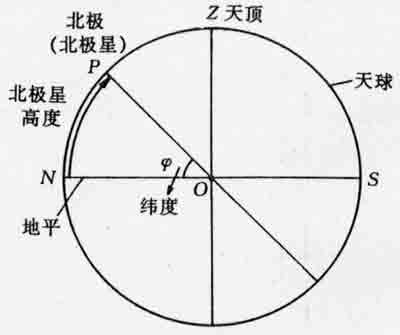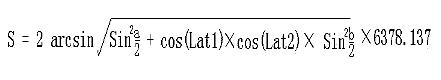# 背景知识# 根据地球上任意两点的经纬度计算两点间的距离

C = sin(MLatA)*sin(MLatB)*cos(MLonA-MLonB) + cos(MLatA)*cos(MLatB)

Distance = R*Arccos(C)*Pi/180

C = sin(LatA)*sin(LatB) + cos(LatA)*cos(LatB)*cos(MLonA-MLonB)

Distance = R*Arccos(C)*Pi/180

C = sin(LatA*Pi/180)*sin(LatB*Pi/180) + cos(LatA*Pi/180)*cos(LatB*Pi/180)*cos((MLonA-MLonB)*Pi/180)

Distance = R*Arccos(C)*Pi/180

C = sin(LatA/57.2958)*sin(LatB/57.2958) + cos(LatA/57.2958)*cos(LatB/57.2958)*cos((MLonA-MLonB)/57.2958)

Distance = R*Arccos(C) = 6371.004*Arccos(C) kilometer = 0.621371192*6371.004*Arccos(C) mile = 3958.758349716768*Arccos(C) milea=latitude1 - latitude2为两点纬度之差 b=longitude1-longitude2 为两点经度之差；
6378.137（KM） 为地球半径

//  时间标识
typedef struct tagDate
{
int                 m_year;         //
int                 m_month;        //
int                 m_day;          //
int                 m_hour;         // ʱ
int                 m_minute;       //
int                 m_second;       //
}GoToDate;

// GPS位置信息
typedef struct tagLocation
{
struct tagDate      m_date;         //  时间
char                        m_status;       //  状态
double                   m_latitude;        //  纬度
double                   m_longitude;   //  经度
char                        m_NS;             //  南北纬度标识
char                        m_EW;            //  东西经度标识
double                 m_speed;        //  速度标识
double                 m_high;         //  高速
}GoToLocation;

//  两坐标之间的距离信息
typedef struct tagPosition
{
double                 m_xdistance;         //  东西经度距离
double                 m_ydistance;         //  南北纬度距离
double                 m_zdistance;         //  高度距离
double                 m_tdistance;         //  坐标直线距离
double                 m_angle;               //  偏角
}GoToPosition;

void ShowPosition(GoToPosition *position)
{
printf("两地水平距离%lf\n", position->m_xdistance);
printf("两地垂直距离%lf\n", position->m_ydistance);
printf("两地高度相差%lf\n", position->m_zdistance);
printf("两地直线距离%lf\n", position->m_tdistance);
printf("两地偏角%lf\n", position->m_angle);
}

const double EARTH_RADIUS = 6378.137;       //地球半径
const double EARTH_RADIUS_C = 6378137; // 赤道半径
const double EARTH_RADIUS_J = 6356725; // 极半径
const double PI = 3.141592654;              // PI

{
return d * PI / 180.0;
}

/*
地球是一个近乎标准的椭球体，
它的赤道半径为6378.140千米，极半径为 6356.755千米，平均半径6371.004千米。

如果我们假设地球是一个完美的球体，那么它的半径就是地球的平均半径，记为R。
如果以0度经线为基 准，那么根据地球表面任意两点的经纬度就可以计算出这两点间的地表距离
（这里忽略地球表面地形对计算带来的误差，仅仅是理论上的估算值）。

设第一点A的经 纬度为(LonA, LatA)，第二点B的经纬度为(LonB, LatB)，按照0度经线的基准，
东经取经度的正值(Longitude)，西经取经度负值(-Longitude)，
北纬取90-纬度值(90- Latitude)，南纬取90+纬度值(90+Latitude)，
则经过上述处理过后的两点被计为(MLonA, MLatA)和(MLonB, MLatB)。
那么根据三角推导，可以得到计算两点距离的如下公式：
C = sin(MLatA)*sin(MLatB)*cos(MLonA-MLonB) + cos(MLatA)*cos(MLatB)

Distance = R*Arccos(C)*Pi/180
*/
/*

πRcosφ/180
π=3.1415926
R=6371 km
φ为纬度
=111.319491*cosφ

= πR/180=111.319491 km
*/
// 获取两个位置点之间的相对距离
// 单位KM
double GetDistance(GoToLocation *left, GoToLocation *right, GoToPosition *position)
{
printf("a = %lf, b = %lf\n", a, b);
//double angle = atan(cos(Rad(right->m_latitude)) * abs(right->m_longitude - left->m_longitude) / abs(right->m_latitude - left->m_latitude));
//printf("%lf\n", angle);
if(position != NULL)
{
printf("***********************************\n");
ShowLocation(left);
ShowLocation(right);
position->m_xdistance = 111.319491 * cos (Rad(left->m_latitude)) * (left->m_longitude - right->m_longitude) / 2;                      // 经度差
position->m_ydistance = 111.319491 * (left->m_latitude - right->m_latitude);                                                     // 维度差
position->m_zdistance = right->m_high -  left->m_high;
position->m_tdistance = s;
position->m_angle = atan(position->m_ydistance / position->m_xdistance) * 180 / PI;
if(position->m_angle < 0)
{
position->m_angle *= -1;
}
/*
position->m_xdistance = 111.319491 * cos (Rad(left->m_latitude)) * b;                      // 经度差
position->m_ydistance = 111.319491 * (a);                                                     // 维度差
position->m_zdistance = right->m_high -  left->m_high;
position->m_tdistance = s;
*/
#ifdef DEBUG
printf("两地水平距离%lf\n", position->m_xdistance);
printf("两地垂直距离%lf\n", position->m_ydistance);
printf("两地高度相差%lf\n", position->m_zdistance);
printf("两地直线距离%lf\n", position->m_tdistance);
printf("两地的偏角%lf\n", position->m_angle);
printf("%lf == %lf", position->m_tdistance, s);
printf("***********************************\n");
#endif
}

return s;
}

int main( )
{
// 经度相同，纬度每差1,相距111.319491
GoToDate     date = {2014, 5, 23, 10, 56, 00};
GoToLocation left = {date,'1', 45.4225, 126.3445, 'N', 'W', 12.00, 20};
GoToLocation right = {date,'1', 44.4211, 126.36455, 'N', 'W', 12.00, 20};
GoToPosition position;
printf("维度差, 垂直%lf\n", 111.319491 * 1);
printf("%lf\n", GetDistance(&left, &right, &position));
ShowPosition(&position);
}

09-0376107-214970
04-282527
10-248867
08-231621
07-11213
06-15
03-181万+
08-311万+
01-14974
09-30
03-131万+
02-202309
05-033324
05-02541
06-156万+
08-095038
05-252406
06-268719